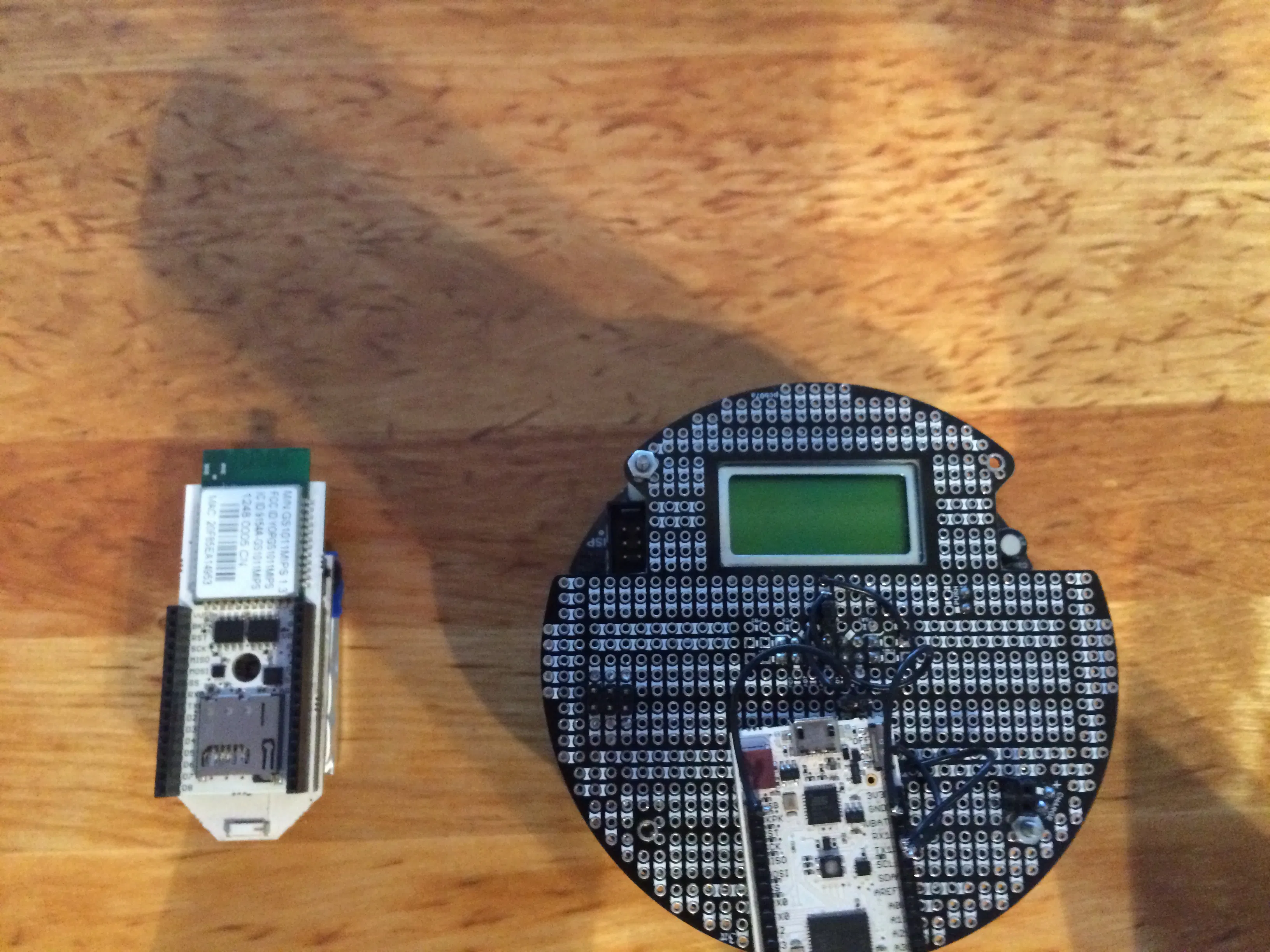Published

# The Web Rover

Control a Pololu Rover through custom scout script, a mobile device accelerometer, and Minecraft

Full instructions provided1,101## Code

### file_5348.txt

C/C++
Type in a caption
```#include "version.h"
#include <SPI.h>
#include <Wire.h>
#include <Scout.h>
#include <GS.h>
#include <bitlash.h>
#include <lwm.h>
#include <js0n.h>

// c0040b2a2f0fcb6f
// http://www.pololu.com/docs/0J21/10.a
enum {
PI_SIGNATURE           = 0x81,
PI_RAW_SENSORS         = 0x86,
PI_CALIBRATED_SENSORS  = 0x87,
PI_TRIMPOT             = 0xB0,
PI_BATTERY_MV          = 0xB1,
PI_PLAY_MUSIC          = 0xB3,
PI_CALIBRATE           = 0xB4,
PI_RESET_CALIBRATION   = 0xB5,
PI_LINE_POSITION       = 0xB6,
PI_CLEAR_LCD           = 0xB7,
PI_PRINT               = 0xB8,
PI_LCD_GOTO_XY         = 0xB9,
PI_AUTOCALIBRATE       = 0xBA,
PI_START_PID           = 0xBB,
PI_STOP_PID            = 0xBC,
PI_M1_FORWARD          = 0xC1,
PI_M1_BACKWARD         = 0xC2,
PI_M2_FORWARD          = 0xC5,
PI_M2_BACKWARD         = 0xC6
};

uint32_t timer = millis();
uint32_t safetyTimer = 0; // start in a disabled state
bool stopped = true;

void setup() {
Scout.setup(SKETCH_NAME, SKETCH_REVISION, SKETCH_BUILD);

initialize3Pi();
}

void loop() {
Scout.loop();
}

void initialize3Pi() {
Serial1.begin(115200);
Serial1.write(PI_SIGNATURE);
Serial1.write(PI_CLEAR_LCD);
Serial1.write(PI_PRINT);
Serial1.write(8);
Serial1.print("Pinoccio");
Serial1.write(PI_LCD_GOTO_XY);
Serial1.write(0);
Serial1.write(1);
Serial1.write(PI_PRINT);
Serial1.write(8);
Serial1.print("Lets go!");
}

numvar moveForward() {
Serial1.write(PI_M1_FORWARD);
Serial1.write(32);
Serial1.write(PI_M2_FORWARD);
Serial1.write(32);
safetyTimer = millis();
stopped = false;
}

numvar turnLeft() {
Serial1.write(PI_M1_BACKWARD);
Serial1.write(32);
Serial1.write(PI_M2_FORWARD);
Serial1.write(32);
safetyTimer = millis();
stopped = false;
}

numvar turnRight() {
Serial1.write(PI_M1_FORWARD);
Serial1.write(32);
Serial1.write(PI_M2_BACKWARD);
Serial1.write(32);
safetyTimer = millis();
stopped = false;
}

numvar moveBackward() {
Serial1.write(PI_M1_BACKWARD);
Serial1.write(32);
Serial1.write(PI_M2_BACKWARD);
Serial1.write(32);
safetyTimer = millis();
stopped = false;
}

numvar stop() {
Serial1.write(PI_STOP_PID);
safetyTimer = millis();
stopped = true;
}
```

### file_5349.html

HTML
```<!doctype html>
<html>
<meta charset="utf-8">
<body>
<script src="https://api.pinocc.io/pinoccio.js"></script>
<script src="js/gyro.min.js"></script>
<script>

var api = pinoccioAPI('96496cq2hj4gngbfa1lnsifjk2');

var s = api.sync({stale:1});
gyro.startTracking(function(o) {
var running = false;
\$("body").html("X: " + o.x + ", Y: " + o.y + "<br>");
if (o.y > 5) {
api.rest({url:"/v1/1/1/command", data:{command:'command.scout(2, "rover.left")'}},function(err,data){
console.log('much win? ',error,data)
});
running = true;
}
if (o.y < -5) {
api.rest({url:"/v1/1/1/command", data:{command:'command.scout(2, "rover.right")'}},function(err,data){
console.log('much win? ',error,data)
});
running = true;
}
if (o.x > 5) {
api.rest({url:"/v1/1/1/command", data:{command:'command.scout(2, "rover.forward")'}},function(err,data){
console.log('much win? ',error,data)
});
running = true;
}
if (o.x < -5) {
api.rest({url:"/v1/1/1/command", data:{command:'command.scout(2, "rover.backward")'}},function(err,data){
console.log('much win? ',error,data)
});
running = true;
}
if (running == false) {
api.rest({url:"/v1/1/1/command", data:{command:'command.scout(2, "rover.stop")'}},function(err,data){
console.log('much win? ',error,data)
});
}
});
</script>
</body>

</html>
```

### file_5351.txt

Lua
```while true do
local running = false
if redstone.getInput("front") == true then
local example = http.post("https://api.pinocc.io/v1/1/1/command", 'command=command.scout(2, "rover.forward")&token={{api token}}')
running = true
end
if redstone.getInput("right") == true then
local example = http.post("https://api.pinocc.io/v1/1/1/command", 'command=command.scout(2, "rover.right")&token={{api token}}')
running = true
end
if redstone.getInput("left") == true then
local example = http.post("https://api.pinocc.io/v1/1/1/command", 'command=command.scout(2, "rover.left")&token={{api token}}')
running = true
end
if redstone.getInput("back") == true then
local example = http.post("https://api.pinocc.io/v1/1/1/command", 'command=command.scout(2, "rover.backward")&token={{api token}}')
running = true
end
if running == false then
local example = http.post("https://api.pinocc.io/v1/1/1/command", 'command=command.scout(2, "rover.stop")&token={{api token}}')
end
end
```